# What Is a Number Line?

#### Video Lesson on What Is a Number Line?

This lesson video Not available at this time available video coming soon

### What Is a Number Line?

A number line is a visual representation of all real numbers. In this lesson, we'll learn how to identify points on a number line. We'll also practice addition and subtraction, letting the number line do all the hard work.

### Learning About Numbers

Let's say you have eight apples. Then I come along and take five of your apples. That's not cool. Maybe you were going to make a pie. Apple theft is a real problem! It's also the way most people begin to learn about numbers. But, it's not the only way. When you're ready to move beyond fruit transactions, you're ready for number lines.

### What Is a Number Line?

A number line is a picture representation of real numbers evenly laid out on a straight line. It looks like this: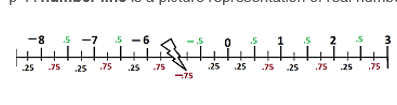Example of a number line

As a reminder, real numbers include just about everything: whole numbers like 7, 15 and 5,832,622; rational numbers like 1/2 and 6.8; and irrational numbers like pi. You could plot any of these on a number line.

What can't you put on a number line? Imaginary numbers, like the square root of -1 and also infinity. You can make your number line as long as you want; you could never find infinity on it. Same goes for numbers you make up, like seventy-twelve.

A number line has a few basic parts. In the middle, there is the origin, which is 0. How can you remember this? Well, in the beginning, there was nothing. I read that somewhere. Also, the origin of most things is nothing. That includes the score at the start of a game or my bank account at the start of a month.

To the right are positive numbers. To the left are negative numbers. Both theoretically go on forever in either direction (though they never reach infinity). It's like the interstate going east and west forever, which is how it seems when you're in the middle of Kansas! Since your screen or paper isn't as big as Kansas, we put arrows on the ends to indicate the line keeps going.

When you draw a number line, you can plot numbers on it however it suits your purpose. Maybe you just want integers between -5 and 5. Maybe you want fractions between -1 and 1. Maybe you want odd numbers. Or, maybe you're like Scrooge Muck calculating his wealth and you want increments in the millions. It's up to you!

### Identifying Points

Sometimes you just need to find a point on a number line. Let's say you were asked to find 4.5 on the line below. Where is it? Well, it's halfway between 4 and 5, right here: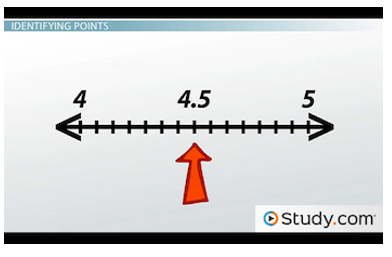4.5 can be found on this number line between 4 and 5.

Or, maybe you'll be asked to identify a point on a line. For example, what is the point below?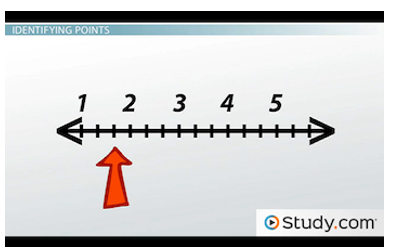You can identify a point on a number line by counting the marks between the numbers.

Okay, notice that there are two marks between each whole number, so each one represents 1/3. This one is 2 marks past 1, so it's 1 and 2/3. This is just like finding points on a ruler; you just need to figure out what each hash mark represents.

### Addition and Subtraction

Numbers lines are particularly useful with addition and subtraction problems. Remember that apple piracy I mentioned? That's a great way to learn subtraction if you happen to have a bunch of apples handy. But, you can do that problem with a number line.

I said you had eight apples. So, find the 8 on the line below. Then, I took five. Since this is subtraction, we go to the left. Why? Because when you subtract, your number's left. Okay, count back 5. We're at 3. So, you have three apples left! That's enough for a tart, right?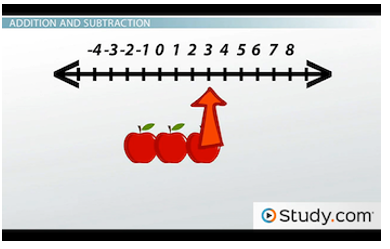You can use a number line for subtraction.

Now, what if I gave you back four apples? Okay, this is addition, so move to the right. Why? Because getting more numbers is just right. Okay, count one, two, three, four. And, we're at 7. I think we're back in pie territory.

### Negative Numbers

Number lines are a great way to work with negative numbers. You can't have -4 apples. I may be an apple thief, but I'm not that good. But, on a number line, you can solve problems involving negatives.

What's -8 + 5? Start at -8 below and move 5 which way? It's addition, so go right. One, two, three, four, five: -3. -8 + 5 = -3.You can use a number line to solve problems that have negative numbers.

What about 2 - 9? Start at 2 and move 9 to the left - for subtraction. One, two, three, four, five, six, seven, eight, nine. We're at -7, so 2 - 9 = -7.

Sometimes, you'll need to bounce back and forth between the positive and negative sides of the origin. But, the principle of what you're doing doesn't change. What is 9 - 12 + 4 - 2? Whoa. This would be a mess with apples. But, we can do it with a number line. Start at 9 below, then move 12 - where? Your number's left, so go left. Now, we're at -3. If we add 4, we go which way? Getting more is right, so go right. Now, we're at positive 1. Then, we go left 2, and we're at -1. So, 9 - 12 + 4 - 2 = -1. With a number line, that wasn't so bad, was it?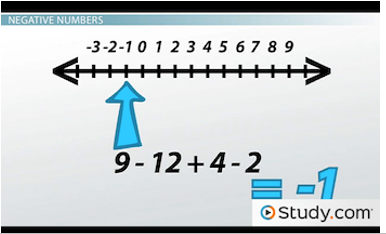Solving 9 - 12 + 4 - 2 on a number line

How about one more? -7 + 6 - 2 + 5. Start at -7 below, then go 6 to the right: we're at -1. Now, 2 to the left: -3. Now, 5 to the right: positive 2. That's it! Just let the number line guide you.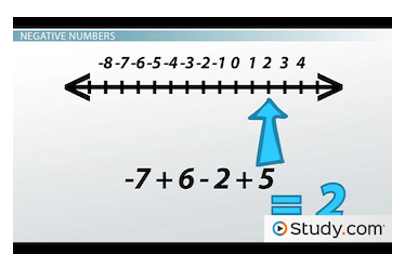Solving -7 + 6 - 2 + 5 on a number line

### Lesson Summary

In summary, a number line is a picture representation of real numbers evenly laid out on a straight line. You can draw the numbers however you'd like. Remember that the middle is the origin, or 0. When you add, you go to the right. When you subtract, you go to the left. Pay attention to what each hash mark represents, and that's all there is to it.

### About us

EntryTest.com is a free service for students seeking successful career.CAT - College of Admission Tests. All rights reserved. College of Admission Tests Online Test Preparation The CAT Online